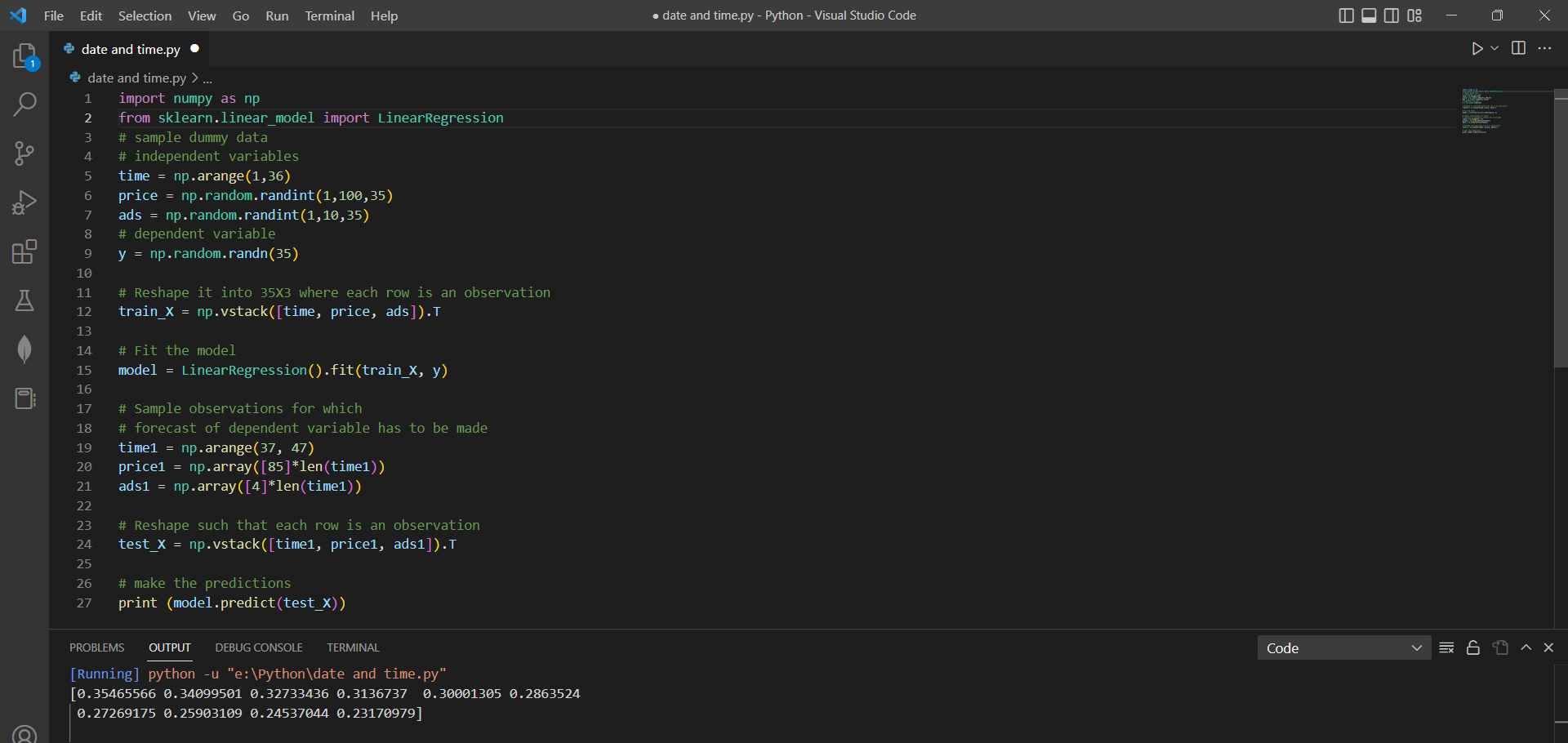# Linear regression model trained to make predictions using the sci-kit-learn library.by vigneshchennai74Updated: Apr 6, 2023Solution Kit

This code demonstrates how a simple linear regression model can be trained and used to make predictions in Python using the sci-kit-learn library. The LinearRegression class from the sklearn.linear_model module in sci-kit-learn is used to build and train linear regression models in Python.

Linear Regression is a supervised machine learning algorithm used for regression problems. In regression problems, the goal is to predict a continuous target variable based on one or more input variables. The linear regression algorithm fits a linear equation to the observed data between the dependent (target) and independent (predictor) variables. The equation is represented by a line that best captures the relationship between the variables.

The model.predict() method in sci-kit-learn's LinearRegression class is used to make predictions for new data based on a trained linear regression model.

Linear Regression is widely used for many applications, including forecasting, modeling, and understanding the relationship between variables.Preview of the output that you will get on running this code from your IDE﻿

### Code

In this solution we have used LinearRegression

1. Copy the code using the "Copy" button above, and paste it in a Python file in your IDE.
2. Run the file to get the output

I hope you found this useful. I have added the link to dependent libraries, version information in the following sections.

I found this code snippet by searching for "use .predict() method in python for Linear regression" in kandi. You can try any such use case!

### Environment Tested

I tested this solution in the following versions. Be mindful of changes when working with other versions.

1. The solution is created in Python 3.7.15 version
2. The solution is tested on sci-kit-learn 1.0.2 version
3. The solution is tested on numpy 1.21.6 version

Using this solution, we are able going to learn how to predict a simple linear regression model using Scikit learn library in Python with simple steps. This process also facilities an easy-to-use, hassle-free method to create a hands-on working version of code which would help use the .predict() method in python for Linear regression in Python.

### Dependent Library

scikit-learnby scikit-learn

Python54584Version:1.2.2scikit-learn: machine learning in Python

Support
Quality
Security
Reuse

scikit-learnby scikit-learn

Python54584Version:1.2.2License: Permissive (BSD-3-Clause)

scikit-learn: machine learning in Python
Support
Quality
Security
Reuse

numpyby numpy

Python23755Version:v1.25.0rc1The fundamental package for scientific computing with Python.

Support
Quality
Security
Reuse

numpyby numpy

Python23755Version:v1.25.0rc1License: Permissive (BSD-3-Clause)

The fundamental package for scientific computing with Python.
Support
Quality
Security